$$\def\loading{......LOADING......Please Wait......} \def\RR{\bf R} \def\real{\mathbb{R}} \def\bold#1{\bf #1} \def\d{\mbox{Cord}} \def\hd{\widehat \mbox{Cord}} \DeclareMathOperator{\cov}{cov} \DeclareMathOperator{\var}{var} \DeclareMathOperator{\cor}{cor} \newcommand{\ac}{\left\{#1\right\}} \DeclareMathOperator{\Ex}{\mathbb{E}} \DeclareMathOperator{\diag}{diag} \newcommand{\bm}{\boldsymbol{#1}} \def\wait{......LOADING......Please Wait......}$$

## What Can We Do with Large Covariance Matrices? Clustering and Regression?

### Xi (Rossi) LUO

University of Texas
Health Science Center
School of Public Health
Dept of Biostatistics
and Data Science
ABCD Research Group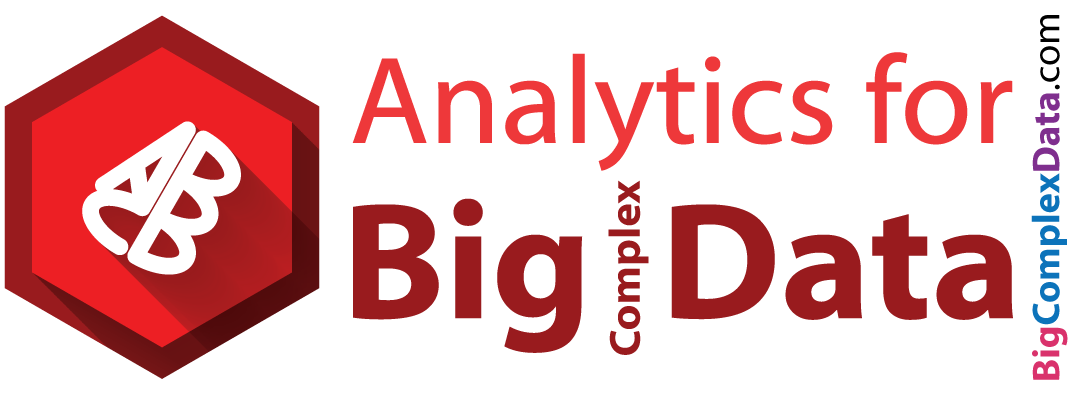HACASA, Houston, Texas
Feburary 12, 2019

Funding: NIH R01EB022911, P20GM103645, P01AA019072, P30AI042853; NSF/DMS (BD2K) 1557467

## Goals

1. Define a global criterion, instead of pair-wise "closeness" criteria, for variable clustering
2. Regress covariance matrix outcomes on vector predictors

# Clustering

## Co-AuthorsFlorentina Bunea
Cornell UniversityChristophe Giraud
Paris Sud University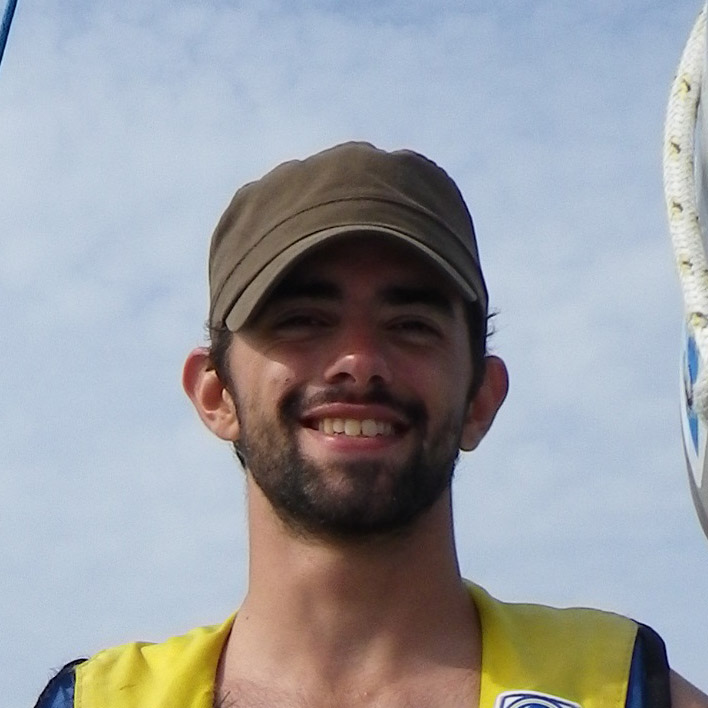Martin Royer
Paris Sud UniversityNicolas Verzelen
INRA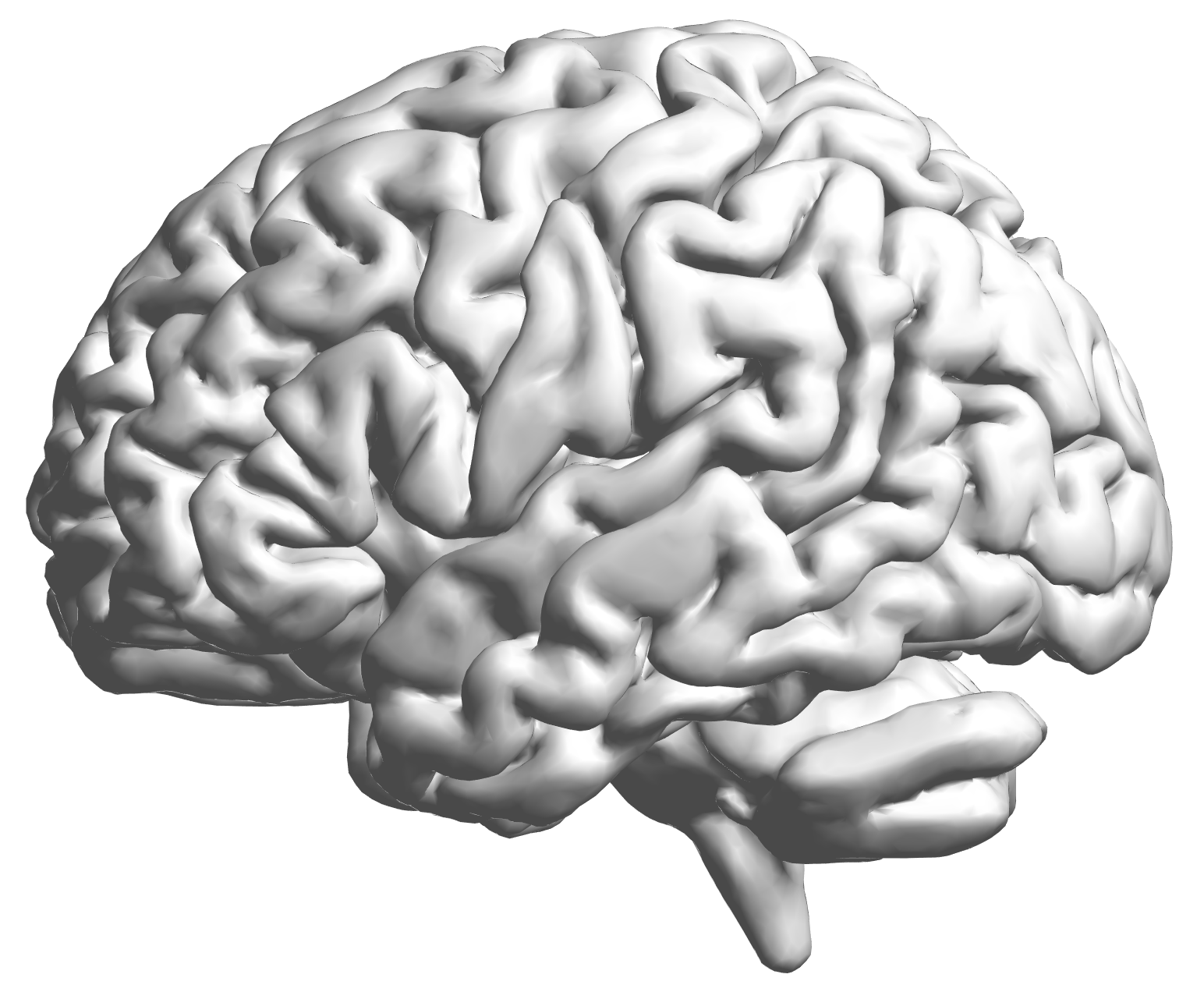fMRI data: blood-oxygen-level dependent (BOLD) signals from each cube/voxel (~millimeters), $10^5$ ~ $10^6$ voxels in total.

## Data Matrix

• Matrix $X_{n \times p}$, all columns standardized
• $n$ time points but temporal correlation removed, like iid
• $p$ voxels but with spatial corraltion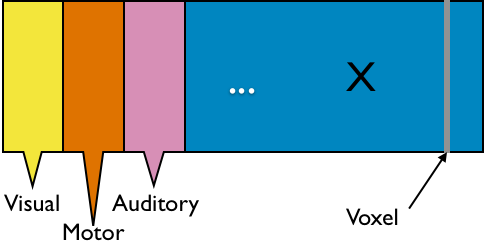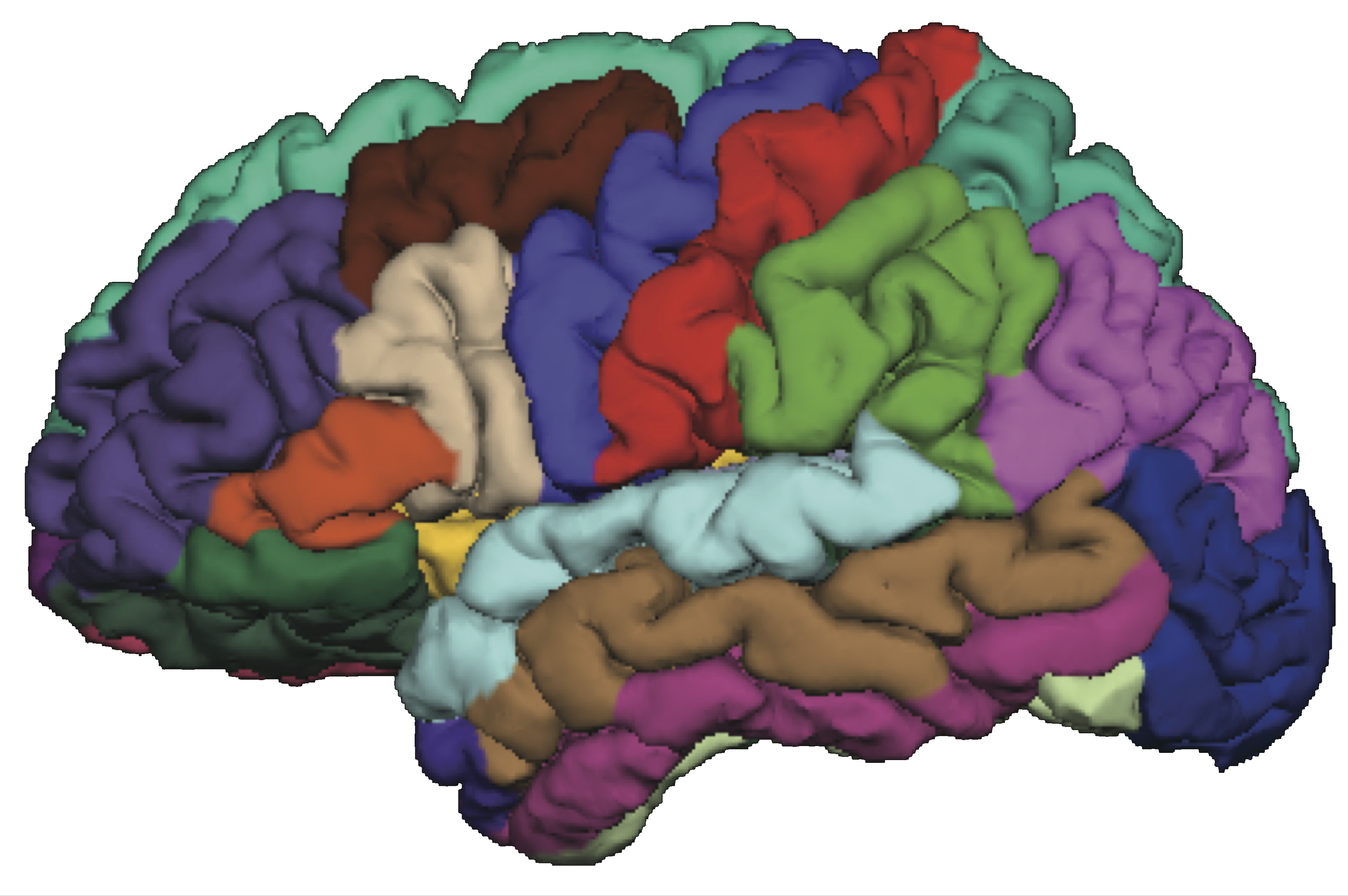• Interested in big spatial networks
• Voxel level: $10^6 \times 10^6$ cov matrix but limited interpretability

## "Network of Networks"

• Hierarchical Covariance Model (a latent var model)
•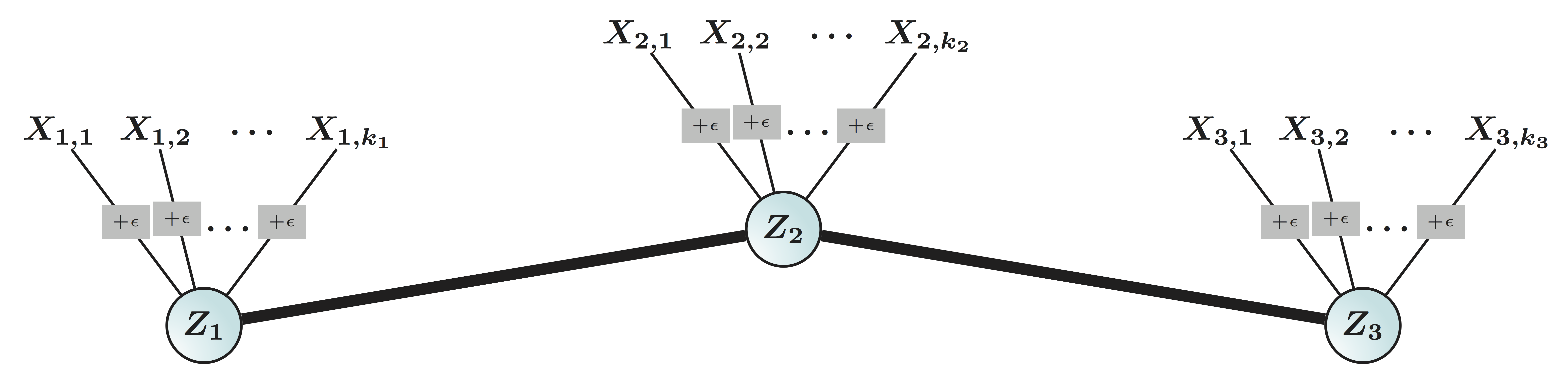• Some ongoing research related to this model
• How to cluster variables together?
• How to estimate cluster signals?
• How to estimate between-cluster connections?
• This talk on how to group(clustering) nodes
• Usually NP-hard and limited theory

# Example

## Example: SP 100 Data

• Daily returns from stocks in SP 100
• Stocks listed in Standard & Poor 100 Indexas of March 21, 2014
• between January 1, 2006 to December 31, 2008
• Each stock is a variable
• Cov/Cor matrices (Pearson's or Kendall's tau)
• Re-order stocks by clusters
• Compare cov patterns with different clustering/ordering

## Cor after Grouping by Clusters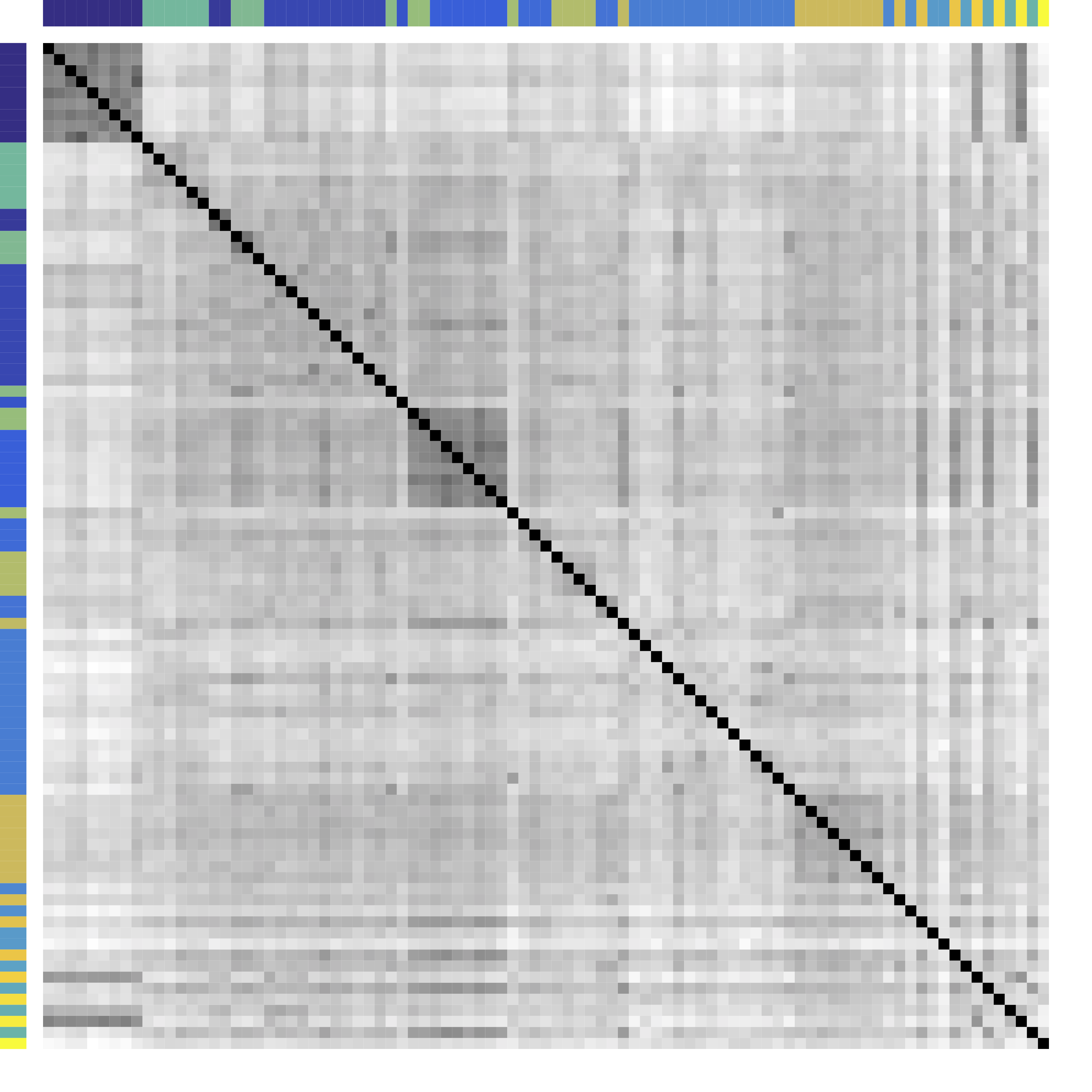Kmeans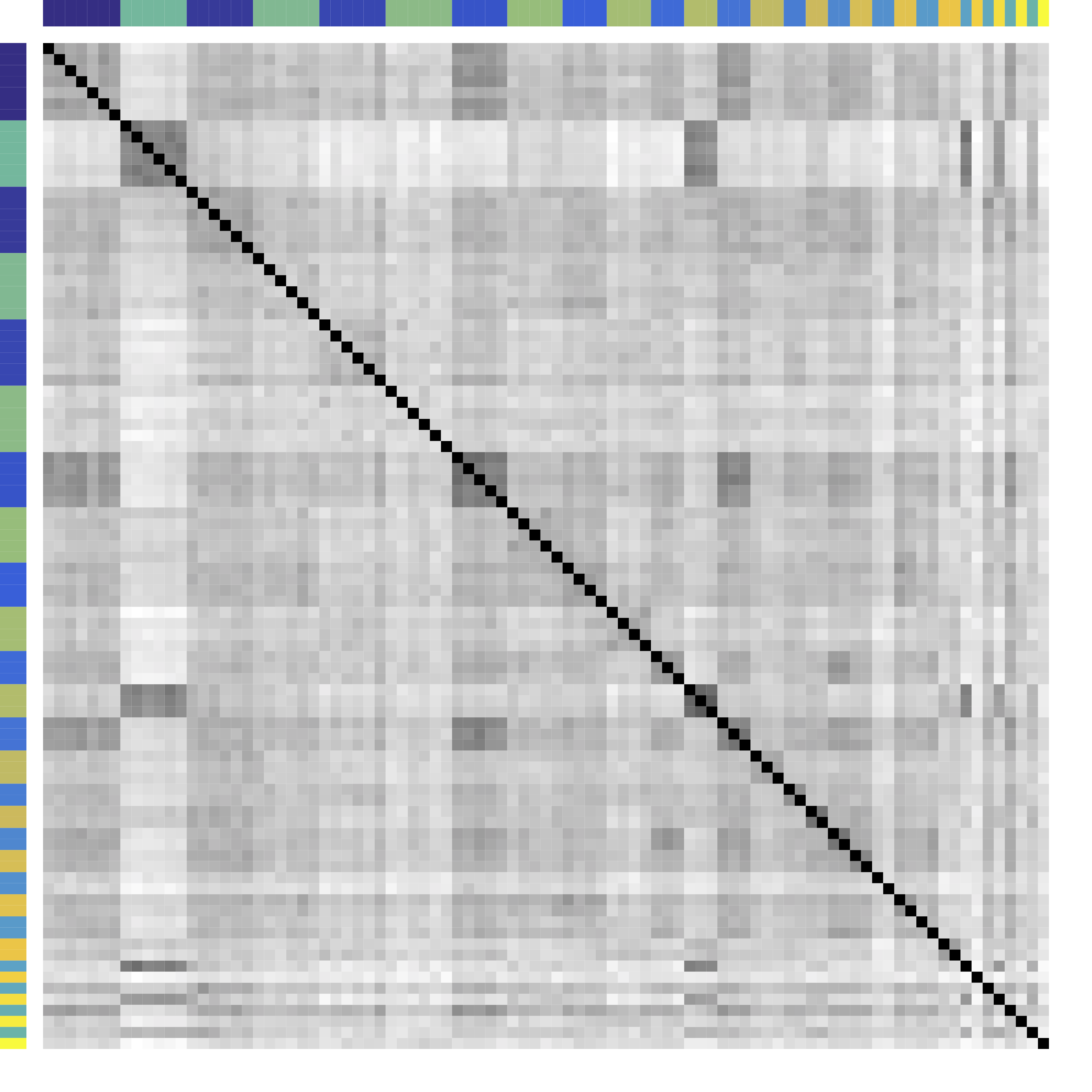Our $G$-models

Ours yields stronger off-diagonal, tile patterns. Black = 1.
Color bars: variable groups/clusters
Off-diagonal: correlations across clusters

## Clustering Results

Industry Ours Kmeans Hierarchical Clustering
Telecom ATT, Verizon ATT, Verizon, Pfizer, Merck, Lilly, Bristol-Myers ATT, Verizon
Railroads Norfolk Southern, Union Pacific Norfolk Southern, Union Pacific Norfolk Southern, Union Pacific, Du Pont, Dow, Monsanto
Home Improvement Home Depot, Lowe’s Home Depot, Lowe’s, Starbucks Home Depot, Lowe’s, Starbucks, Costco, Target, Wal-Mart, FedEx, United Parcel Service
$\cdots$
All methods yield 30 clusters.

# Model

## Problem

• Let ${X} \in \real^p$ be a zero mean random vector
• Divide variables into partitions/clusters
• Example: $\{ \{X_1, X_3, X_7\}, \{X_2, X_5\}, \dotsc \}$
• Theoretical: define uniquely identifiable partition $G$ such that all $X_a$ in $G_k$ are statistically "similar"
• DS: find "helpful" partition that show cov patterns

## Related Methods

• Clustering: Kmeans and hierarchical clustering
• Limitations: low signal-noise-ratio, theory

## Kmeans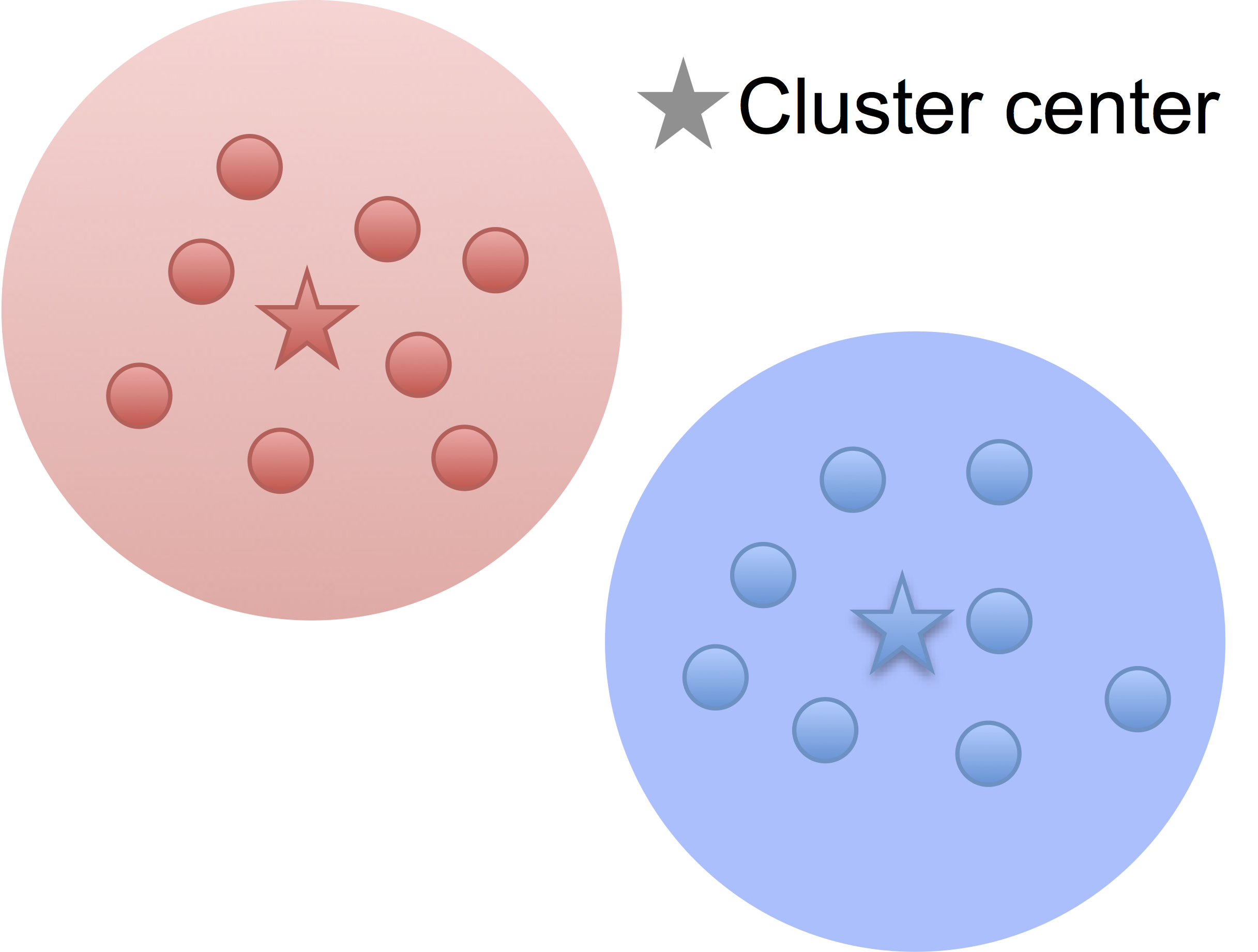Low noise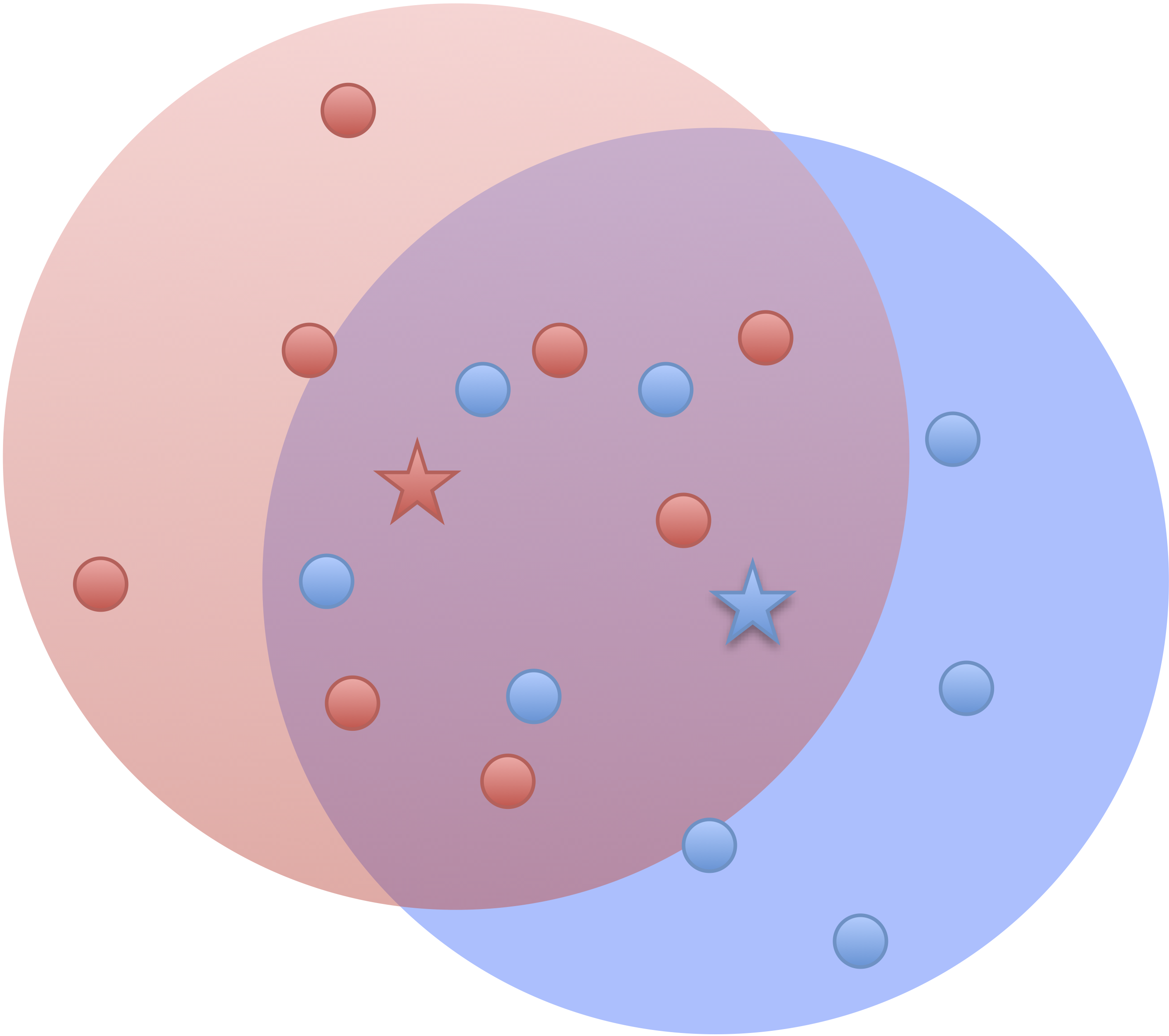High noise

• Cluster points together if pairwise distance small
• Clustering accuracy depends on the noise

## Kmeans: Generative Model

• Data $X_{n\times p}$: $p$ variables from partition $G$: $$G=\{ \{X_1, X_3, X_7\}, \{X_2, X_5\}, \dotsc \}$$
• Mixture Gaussian: if variable $X_j \in \real^n$ comes from cluster $G_k$ Hartigan, 1975 $$X_{j} = Z_k + \epsilon_j, \quad Z_k \bot \epsilon_j$$
• Kmeans minimizes over $G$ (and centroid $Z$): $$\sum_{k=1}^K \sum_{j\in G_k} \left\| X_j - Z_k \right\|_2^2$$
Strictly, Kmeans considers data points in $\real^p$ from $K$ populations

## $G$-Latent Cov

• We call $G$-latent model: $$X_{j} = Z_k + \epsilon_j, \quad Z_k \bot \epsilon_j \mbox{ and } j\in G_k$$
• WLOG, all variables are standardized
• Intuition: variables $j\in G_k$ form net communitiesLuo, 2014

## Matrix Representation

$$X_{n\times p}=\underbrace{Z_{n\times k}}_\text{Source/Factor} \quad \underbrace{G_{k\times p}}_\text{Mixing/Loading} + \underbrace{E_{n\times p}}_{Error} \qquad Z \bot E$$

• Clustering: $G$ is $0/1$ matrix for $k$ clusters/ROIs
• Decomposition: under conditions
• PCA/factor analysis: orthogonality
• ICA: orthogonality → independence
• matrix decomposition: e.g. non-negativity• Our model identifiable even if $\cor(Z_1, Z_2) \ne 0$
• Two brain clusters red/blue talk to each other
• Identifiable if "$\cor(Z_1, Z_2) \gt \var(Z_1)\gt \var(Z_2)$"
• Other models identifiable usually if $\cor(Z_1, Z_2) = 0$

## Principals Behind Other Clustering

• The Euclidean distance for hierarchical clustering and Kmeans, for two columns/voxles $X_a$ and $X_b$: $$\|X_a - X_b \|_2^2 = 2(1-\cor(X_a, X_b))$$
• Cluster $a$ and $b$ based on these two variables only, and this is NOT what we will consider in $G$-models
• Recall $X_i = Z_k + E_i$ $i \in G_k$
• Cor depends mainly on $\var(E)$ if SNR is low
• Distance
• larger even if generated by same $Z$ and large error
• smaller even if generated by different $Z$ and small error
• Worse, clusters close because of correlated $Z$

# Generalization: Latent Var $\rightarrow$ Block Cov

## Example: $G$-Block

• Set $G=\ac{\ac{1,2};\ac{3,4,5}}$, $X \in \real^p$ has $G$-block cov
$$\Sigma =\left(\begin{array}{ccccc} {\color{red} D_1} & {\color{red} C_{11} }&C_{12} & C_{12}& C_{12}\\ {\color{red} C_{11} }&{\color{red} D_1 }& C_{12} & C_{12}& C_{12} \\ C_{12} & C_{12} &{\color{green} D_{2}} & {\color{green} C_{22}}& {\color{green} C_{22}}\\ C_{12} & C_{12} &{\color{green} C_{22}} &{\color{green} D_2}&{\color{green} C_{22}}\\ C_{12} & C_{12} &{\color{green} C_{22}} &{\color{green} C_{22}}&{\color{green} D_2} \end{array}\right)$$
• Matrix math: $\Sigma = G^TCG + d$
• We allow $|C_{11} | \lt | C_{12} |$ or $C \prec 0$
• Kmeans/HC leads to block-diagonal cor matrices (permutation)
• Clustering based on $G$-Block
• Generalizing $G$-Latent which requires $C\succ 0$

## Minimum $G$ Partition

Theorem: $G^{\beta}(X)$ is the minimal partition induced by $a\stackrel{G^{\beta}}{\sim} b$
iff $\var(X_{a})=\var(X_{b})$ and $\cov(X_{a},X_{c})=\cov(X_{b},X_{c})$ for all $c\neq a,b$. Moreover, if the matrix of covariances $C$ corresponding to the partition $G(X)$ is positive-semidefinite, then this is the unique minimal partition according to which ${X}$ admits a latent decomposition.

The minimal partition is unique under regularity conditions.

# Method

## New Metric: CORD

• First, pairwise correlation distance (like Kmeans)
• Gaussian copula: $$Y:=(h_1(X_1),\dotsc,h_p(X_p)) \sim N(0,R)$$
• Let $R$ be the correlation matrix
• Gaussian: Pearson's
• Gaussian copula: Kendall's tau transformed, $R_{ab} = \sin (\frac{\pi}{2}\tau_{ab})$
• Second, maximum difference of correlation distances $$\d(a,b) := \max_{c\neq a,b}|R_{ac}-R_{bc}|$$
• Third, group variables $a$, $b$ together if $\d(a,b) = 0$

## The enemy of my enemy is my friend!

Image credit: http://sutherland-careers.com/

## Algorithm: Main Idea

• Greedy: one cluster at a time, avoiding NP-hard
• Cluster variables together if CORD metric $$\max_{c\neq a,b}|\hat{R}_{ac}-\hat{R}_{bc}| \lt \alpha$$ where $\alpha$ is a tuning parameter
• $\alpha$ is chosen by theory or CV

# Theory

## Condition

Let $\eta \geq 0$ be given. Let ${ X}$ be a zero mean random vector with a Gaussian copula distribution with parameter $R$. $$\begin{multline} \mathcal{R}(\eta) := \{R: \ \d(a,b) := \max_{c\neq a,b}|R_{ac}-R_{bc}|>\eta\quad \\ \textrm{for all}\ a\stackrel{G(X)}{\nsim}b.\} \end{multline}$$ Group separation condition: $R \in \mathcal{R}(\eta)$.

The signal strength $\eta$ need to be large.

## Consistency

Theorem: Define $\tau=|\widehat R-R|_{\infty}$ and we consider two parameters $(\alpha,\eta)$ fulfilling $$\begin{equation} \alpha\geq 2\tau\quad\textrm{and}\quad \eta\geq2\tau+\alpha. \end{equation}$$ Then, applying our algorithm we have $\widehat G=G(X)$ whp.

Ours recovers exact clustering with high probability.

## Minimax

Theorem: $P_{\Sigma}$ the likelihood based on $n$ independent observations of ${ X} \stackrel{d}{=} \mathcal{N}(0,\Sigma)$. For any \begin{equation}\label{etamin} 0\leq \eta \lt \eta^{*}:={\sqrt{\log(p(p-1)/2)}\over \sqrt{(2+e^{-1})n} +\sqrt{\log(p(p-1)/2)}}\,, \end{equation} we have $$\inf_{\widehat G}\sup_{R \in \mathcal{R}(\eta)} P_{\Sigma}(\widehat G\neq G^{\beta}(X))\geq {1\over 2e+1}\geq {1\over 7} \,,$$ where the infimum is taken over all possible estimators.

Our method is minimax optimal.

## Choosing Number of Clusters

• Split data into 3 parts
• Use part 1 of data to estimate clusters $\hat{G}$ for each $\alpha$
• Use part 2 to compute between variable difference $$\delta^{(2)}_{ab} = R_{ac}^{(2)} - R_{bc}^{(2)}, \quad c \ne a, b.$$
• Use part 3 to generate "CV" loss $$\mbox{CV}(\hat{G}) = \sum_{a \lt b} \| \delta^{(3)}_{ab} - \delta^{(2)}_{ab} 1\{ a \mbox{ not clustered w/ } b \} \|^2_\infty.$$
• Pick $\alpha$ with the smallest loss above

## Theory for CV

Theorem: If either: (i) $X$ is sub-Gaussian with correlation matrix $R$; or (ii) $X$ has a copula distribution with copula correlation matrix $R$, then we have $E[\mbox{CV}(G^*)] \lt E[\mbox{CV}(G)]$, for any $G\ne G^*$.
Theoretical support for selecting $G^*$ from data.

# Simulations

## Setup

• Generate from various $C$: block, sparse, negative
• Compare:
• Exact recovery of groups (theoretical tuning parameter)
• Cross validation (data-driven tuning parameter)
• Cord metric vs (semi)parametetric cor (regardless of tuning)

## Exact Recovery

Different models for $C$="$\cov(Z)$" and $\alpha = 2 n^{-1/2} \log^{1/2} p$

Vertical lines: theoretical sample size based on our lower bound

HC and Kmeans fail even if inputting the true $K$.

## Cross Validation

Recovery % in red and CV loss in black.

CV selects the constants to yield close to 100% recovery, as predicted by our theory (at least for large $n>200$)

# Real Data

## fMRI Studies

Sub 1, Sess 1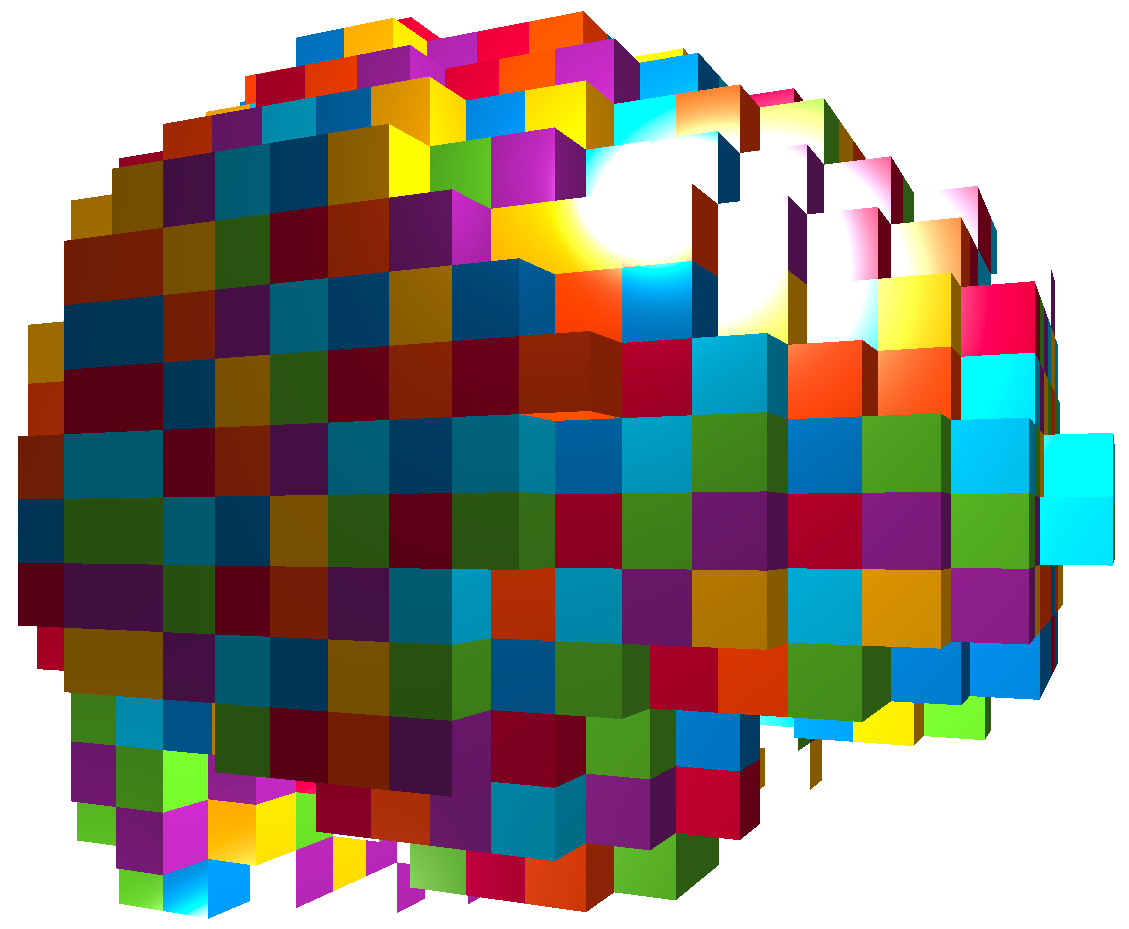Time 12~200

Sub i, Sess jSub ~100, Sess ~4This talk: one subject, two sessions (to test replicability)

## Functional MRI

• fMRI matrix: BOLD from different brain regions
• Variable: different brain regions
• Sample: time series (after whitening or removing temporal correlations)
• Clusters of brain regions
• Two data matrices from two scan sessions OpenfMRI.org
• Use Power's 264 regions/nodes

## Test Prediction/Reproducibilty

• Find partitions using the first session data
• Average each block cor to improve estimation
• Compare with the cor matrix from the second scan $$\| Avg_{\hat{G}}(\hat{\Sigma}_1) - \hat{\Sigma}_2 \|$$ where we used $\hat{G}$ to do block-averaging.
• Difference is smaller if clustering $\hat{G}$ is better

Vertical lines: fixed (solid) and data-driven (dashed) thresholds

Smaller replication difference for almost all $K$## Discussion

• Cov + clustering = Connectivity + ROI
• Identifiability, accuracy, optimality
• $G$-models: $G$-latent, $G$-block, $G$-exchangeable
• New metric, method, and theory
• Paper: google "cord clustering"(arXiv 1508.01939)
• To appear in Annals of Statistics, 2019
• R package: cord on CRAN

# Matix Regression

## Co-Authors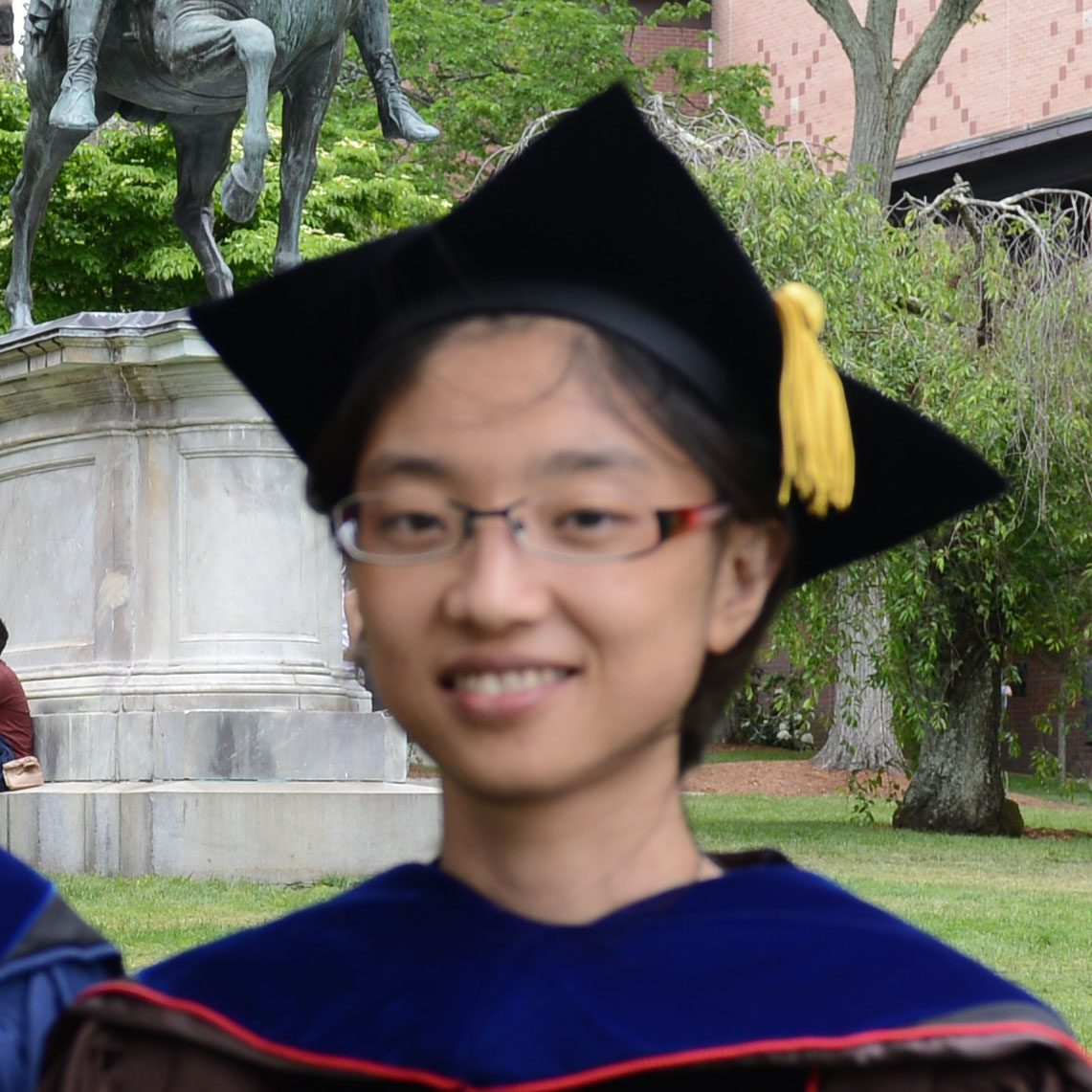Yi Zhao
Johns Hopkins BiostatBingkai Wang
Johns Hopkins BiostatStewart Mostofsky
Johns Hopkins MedicineBrian Caffo
Johns Hopkins Biostat

## Motivating Example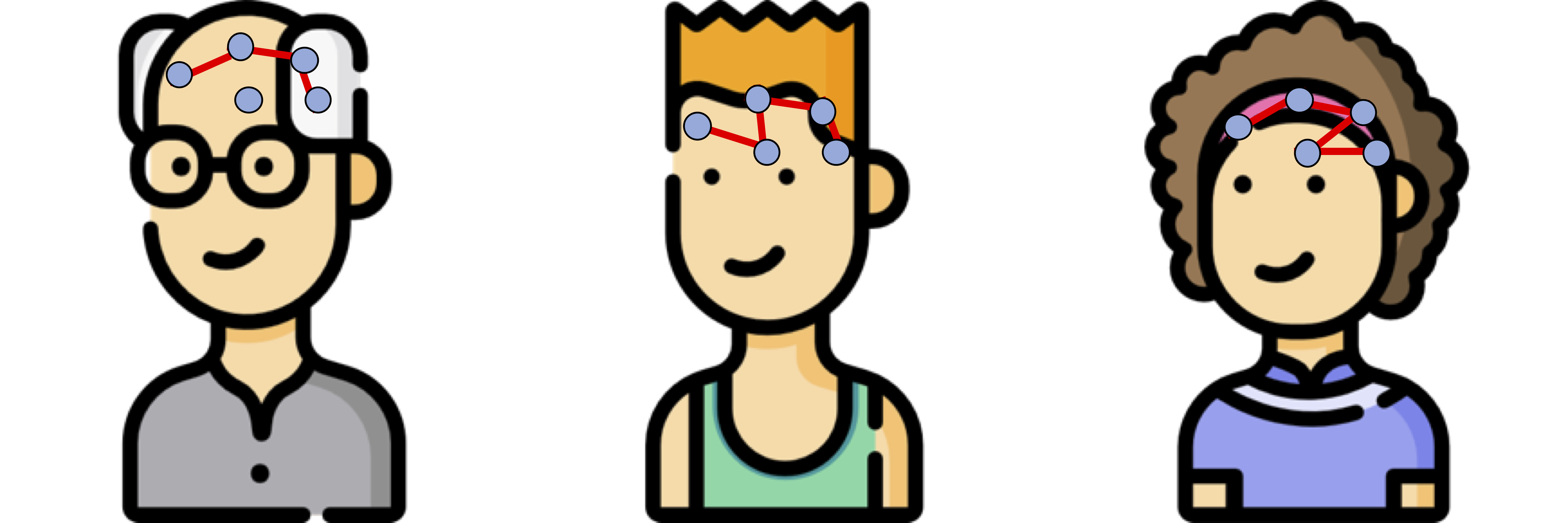Brain network connections vary by covariates (e.g. age/sex)

Goal: model how covariates change network connections

$$\textrm{function}(\textbf{graph}) = \textbf{age}\times \beta_1 + \textbf{sex}\times \beta_2 + \cdots$$

## Resting-state fMRI Networks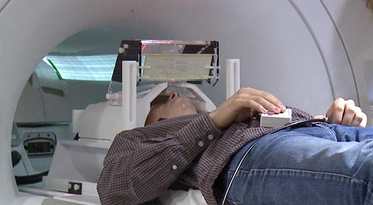• fMRI measures brain activities over time
• Resting-state: "do nothing" during scanning

• Brain networks constructed using cov/cor matrices of time series## Mathematical Problem

• Given $n$ (semi-)positive matrix outcomes, $\Sigma_i\in \real^{p\times p}$
• Given $n$ corresponding vector covariates, $x_i \in \real^{q}$
• Find function $g(\Sigma_i) = x_i \beta$, $i=1,\dotsc, n$
• In essense, regress matrices on vectors

## Some Related Problems

• Heterogeneous regression or weighted LS:
• Usually for scalar variance $\sigma_i$, find $g(\sigma_i) = f(x_i)$
• Goal: to improve efficiency, not to interpret $x_i \beta$
• Covariance models Anderson, 73; Pourahmadi, 99; Hoff, Niu, 12; Fox, Dunson, 15; Zou, 17
• Model $\Sigma_i = g(x_i)$, sometimes $n=i=1$
• Goal: better models for $\Sigma_i$
• Multi-group PCA Flury, 84, 88; Boik 02; Hoff 09; Franks, Hoff, 16
• No regression model, cannot handle vector $x_i$
• Goal: find common/uncommon parts of multiple $\Sigma_i$
• Ours: $g(\Sigma_i) = x_i \beta$, $g$ inspired by PCA

## Massive Edgewise Regressions

• Intuitive method by mostly neuroscientists
• Try $g_{j,k}(\Sigma_i) = \Sigma_{i}[j,k] = x_i \beta$
• Repeat for all $(j,k) \in \{1,\dotsc, p\}^2$ pairs
• Essentially $O(p^2)$ regressions for each connection
• Limitations: multiple testing $O(p^2)$, failure to accout for dependencies between regressions

# Model and Method

## Model

• Find principal direction (PD) $\gamma \in \real^p$, such that: $$\log({\gamma}^\top\Sigma_{i}{\gamma})=\beta_{0}+x_{i}^\top{\beta}_{1}, \quad i =1,\dotsc, n$$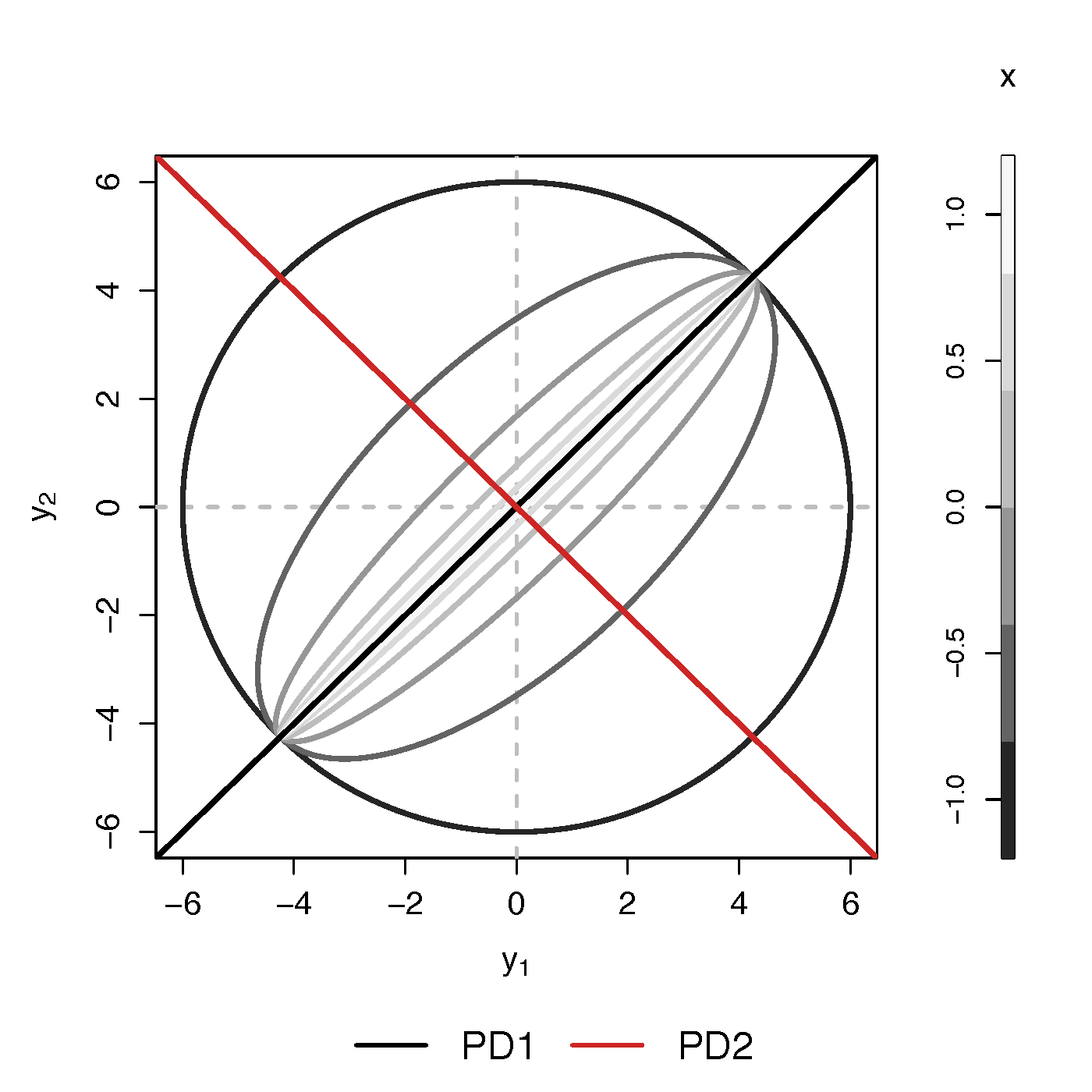Example (p=2): PD1 largest variation but not related to $x$

PCA selects PD1, Ours selects PD2

• Scalability: potentially for $p \sim 10^6$ or larger
• Interpretation: covariate assisted PCA
• Turn unsupervised PCA into supervised
• Sensitivity: target those covariate-related variations
• Covariate assisted SVD?
• Applicability: other big data problems besides fMRI

## Method

• MLE with constraints: $$\scriptsize \begin{eqnarray}\label{eq:obj_func} \underset{\boldsymbol{\beta},\boldsymbol{\gamma}}{\text{minimize}} && \ell(\boldsymbol{\beta},\boldsymbol{\gamma}) := \frac{1}{2}\sum_{i=1}^{n}(x_{i}^\top\boldsymbol{\beta}) \cdot T_{i} +\frac{1}{2}\sum_{i=1}^{n}\boldsymbol{\gamma}^\top \Sigma_{i}\boldsymbol{\gamma} \cdot \exp(-x_{i}^\top\boldsymbol{\beta}) , \nonumber \\ \text{such that} && \boldsymbol{\gamma}^\top H \boldsymbol{\gamma}=1 \end{eqnarray}$$
• Two obvious constriants:
• C1: $H = I$
• C2: $H = n^{-1} (\Sigma_1 + \cdots + \Sigma_n)$

## Choice of $H$

Proposition: When (C1) $H=\boldsymbol{\mathrm{I}}$ in the optimization problem, for any fixed $\boldsymbol{\beta}$, the solution of $\boldsymbol{\gamma}$ is the eigenvector corresponding to the minimum eigenvalue of matrix $$\sum_{i=1}^{n}\frac{\Sigma_{i}}{\exp(x_{i}^\top\boldsymbol{\beta})}$$

Will focus on the constraint (C2)

## Algoirthm

• Iteratively update $\beta$ and then $\gamma$
• Extension to multiple $\gamma$:
• After finding $\gamma^{(1)}$, we will update $\Sigma_i$ by removing its effect
• Search for the next PD $\gamma^{(k)}$, $k=2, \dotsc$
• Impose the orthogonal constraints such that $\gamma^{k}$ is orthogonal to all $\gamma^{(t)}$ for $t\lt k$

## Theory for $\beta$

Theorem: Assume $\sum_{i=1}^{n}x_{i}x_{i}^\top/n\rightarrow Q$ as $n\rightarrow\infty$. Let $T=\min_{i}T_{i}$, $M_{n}=\sum_{i=1}^{n}T_{i}$, under the true $\boldsymbol{\gamma}$, we have \begin{equation} \sqrt{M_{n}}\left(\hat{\boldsymbol{\beta}}-\boldsymbol{\beta}\right)\overset{\mathcal{D}}{\longrightarrow}\mathcal{N}\left(\boldsymbol{\mathrm{0}},2 Q^{-1}\right),\quad \text{as } n,T\rightarrow\infty, \end{equation} where $\hat{\boldsymbol{\beta}}$ is the maximum likelihood estimator when the true $\boldsymbol{\gamma}$ is known.

## Theory for $\gamma$

Theorem: Assume $\Sigma_{i}=\Gamma\Lambda_{i}\Gamma^\top$, where $\Gamma=(\boldsymbol{\gamma}_{1},\dots,\boldsymbol{\gamma}_{p})$ is an orthogonal matrix and $\Lambda_{i}=\mathrm{diag}\{\lambda_{i1},\dots,\lambda_{ip}\}$ with $\lambda_{ik}\neq\lambda_{il}$ ($k\neq l$), for at least one $i\in\{1,\dots,n\}$. There exists $k\in\{1,\dots,p\}$ such that for $\forall~i\in\{1,\dots,n\}$, $\boldsymbol{\gamma}_{k}^\top\Sigma_{i}\boldsymbol{\gamma}_{k}=\exp(x_{i}^\top\boldsymbol{\beta})$. Let $\hat{\boldsymbol{\gamma}}$ be the maximum likelihood estimator of $\boldsymbol{\gamma}_{k}$ in Flury, 84. Then assuming that the assumptions are satisfied, $\hat{ \boldsymbol{\beta}}$ from our algorithm is $\sqrt{M_{n}}$-consistent estimator of $\boldsymbol{\beta}$.

# Simulations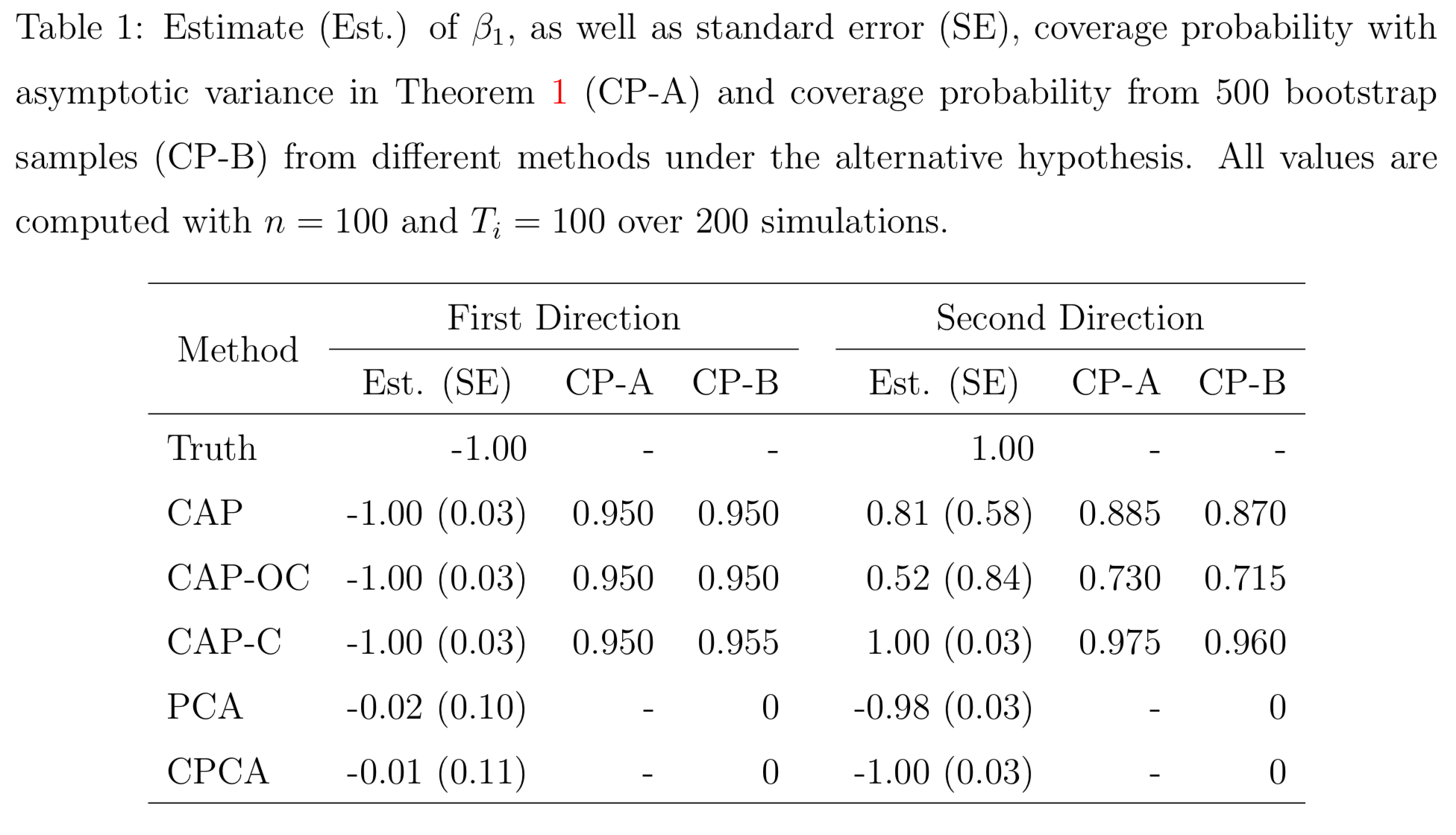PCA and common PCA do not find the first principal direction, because they don't model covariates

# Resting-state fMRI

## Regression CoefficientsAge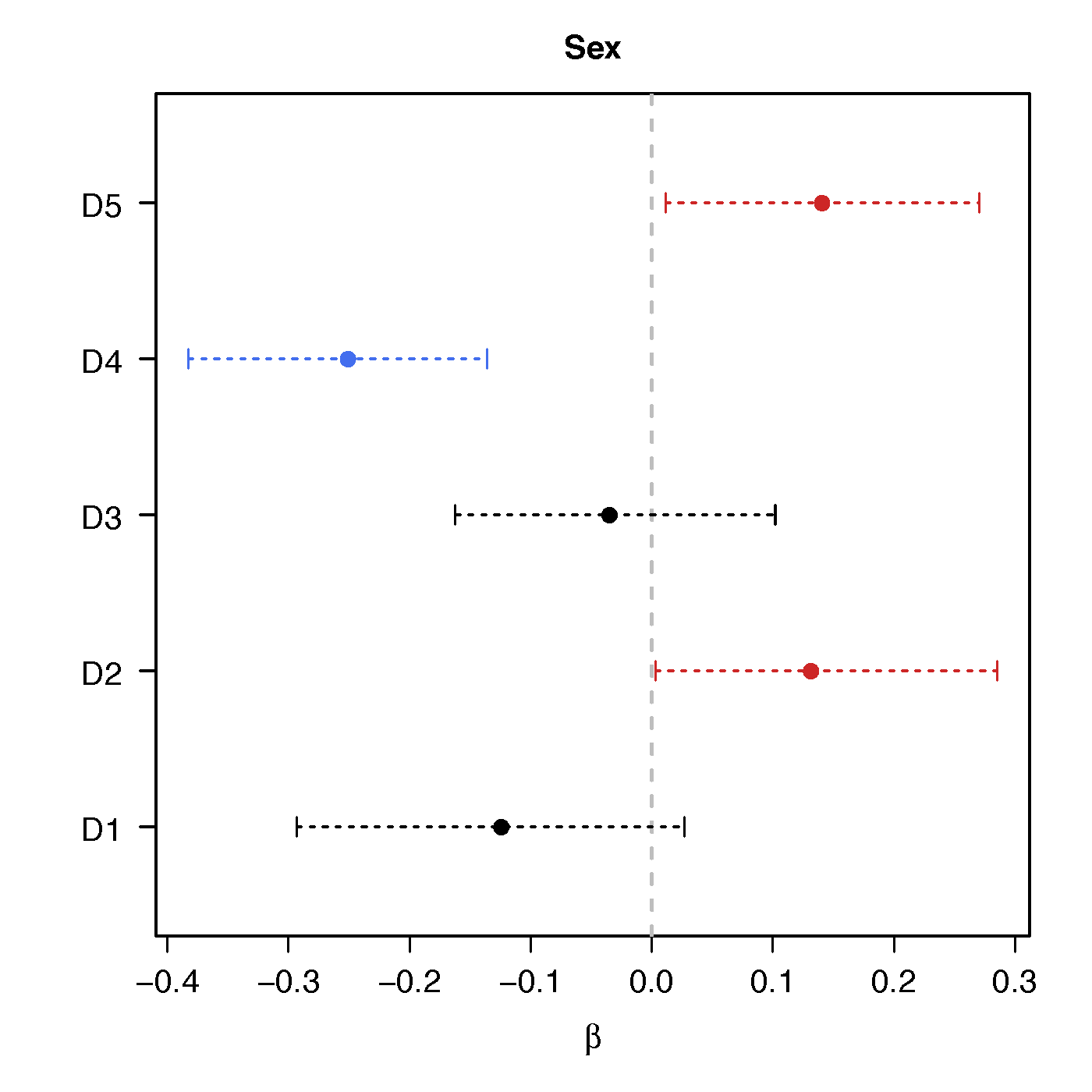Sex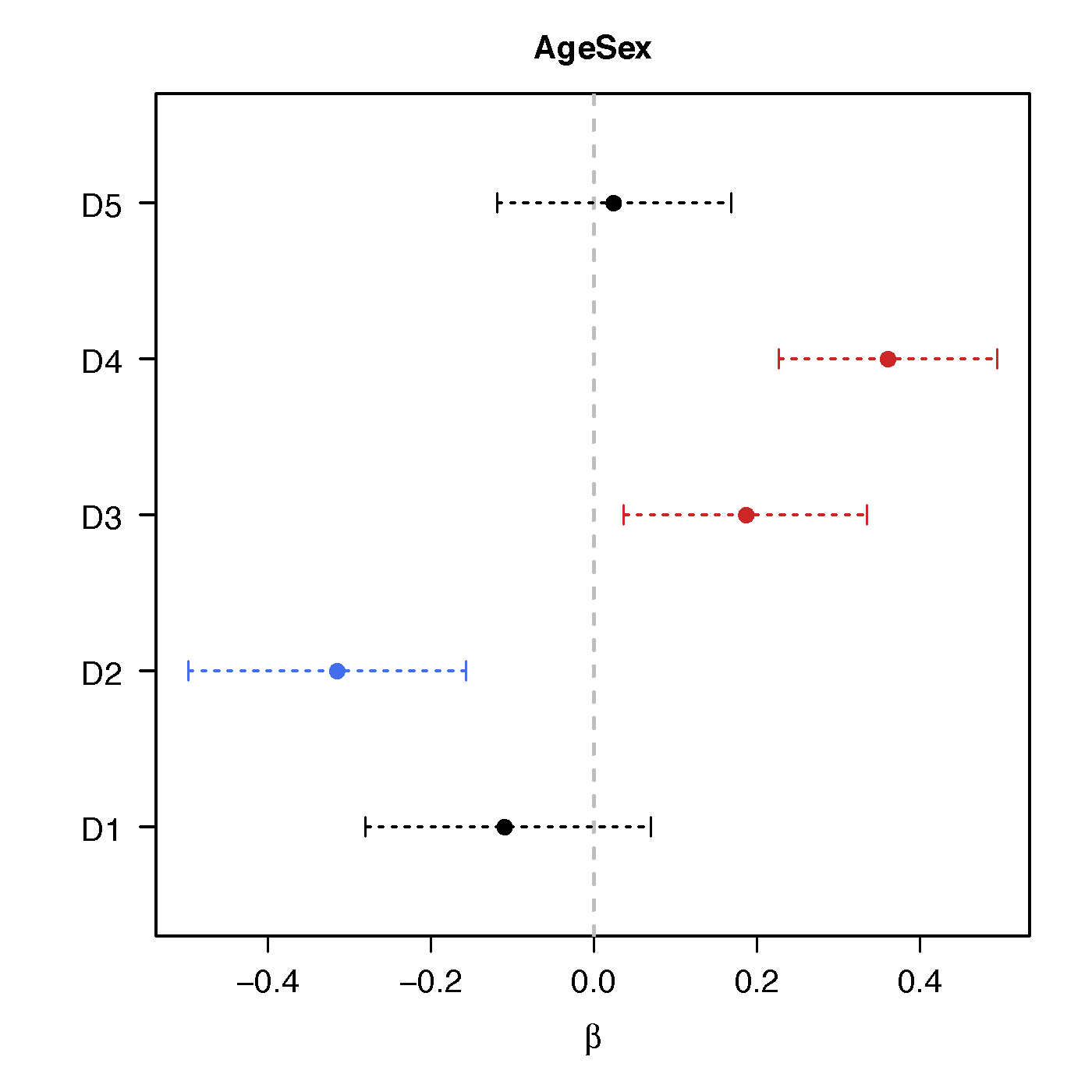Age*Sex

No statistical significant changes were found by massive edgewise regression

## Brain Map of $\gamma$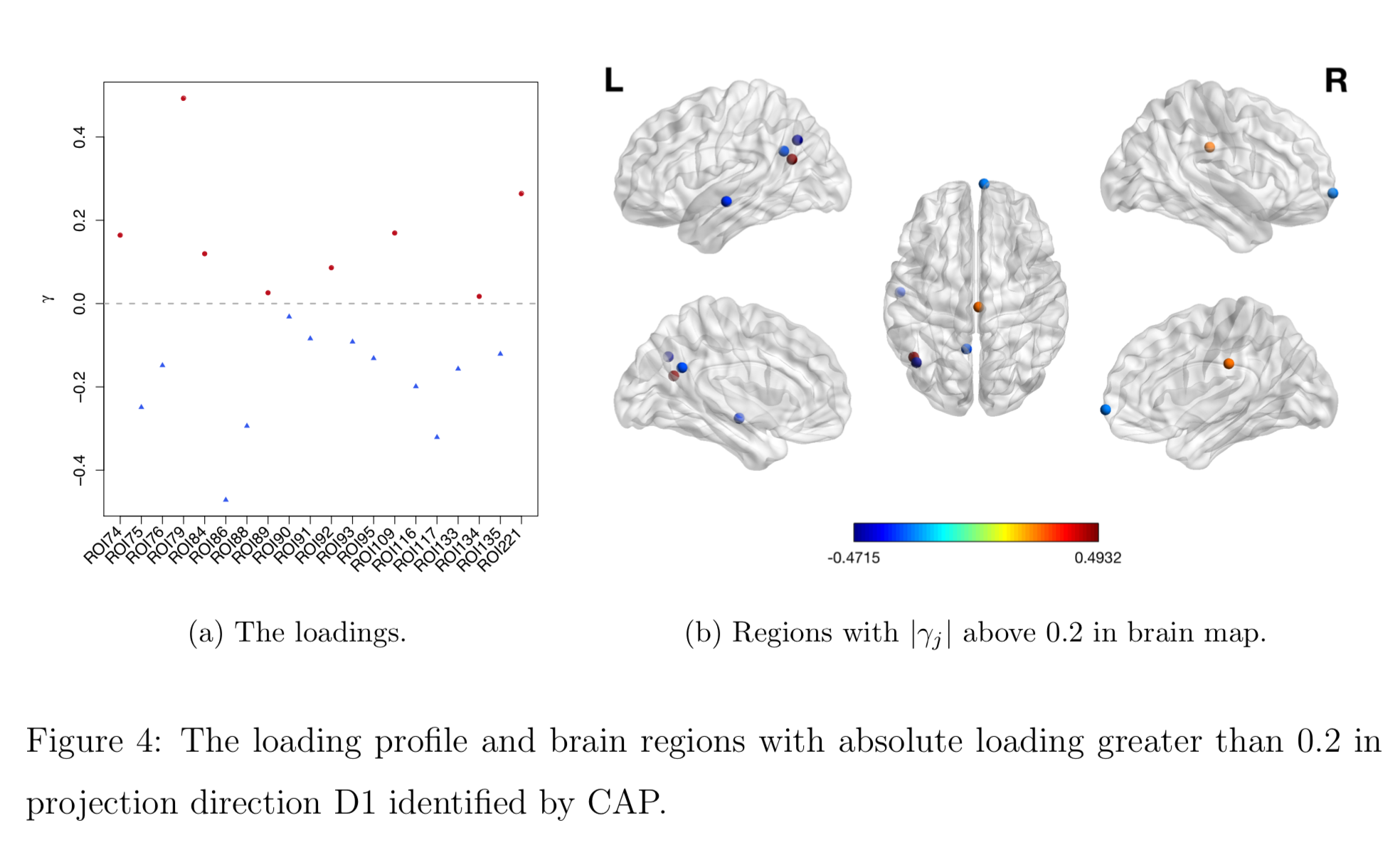## Discussion

• Regress matrices on vectors
• Method to identify covariate-related directions
• Theorectical justification
• Manuscript: DOI: 10.1101/425033
• R pkg: cap